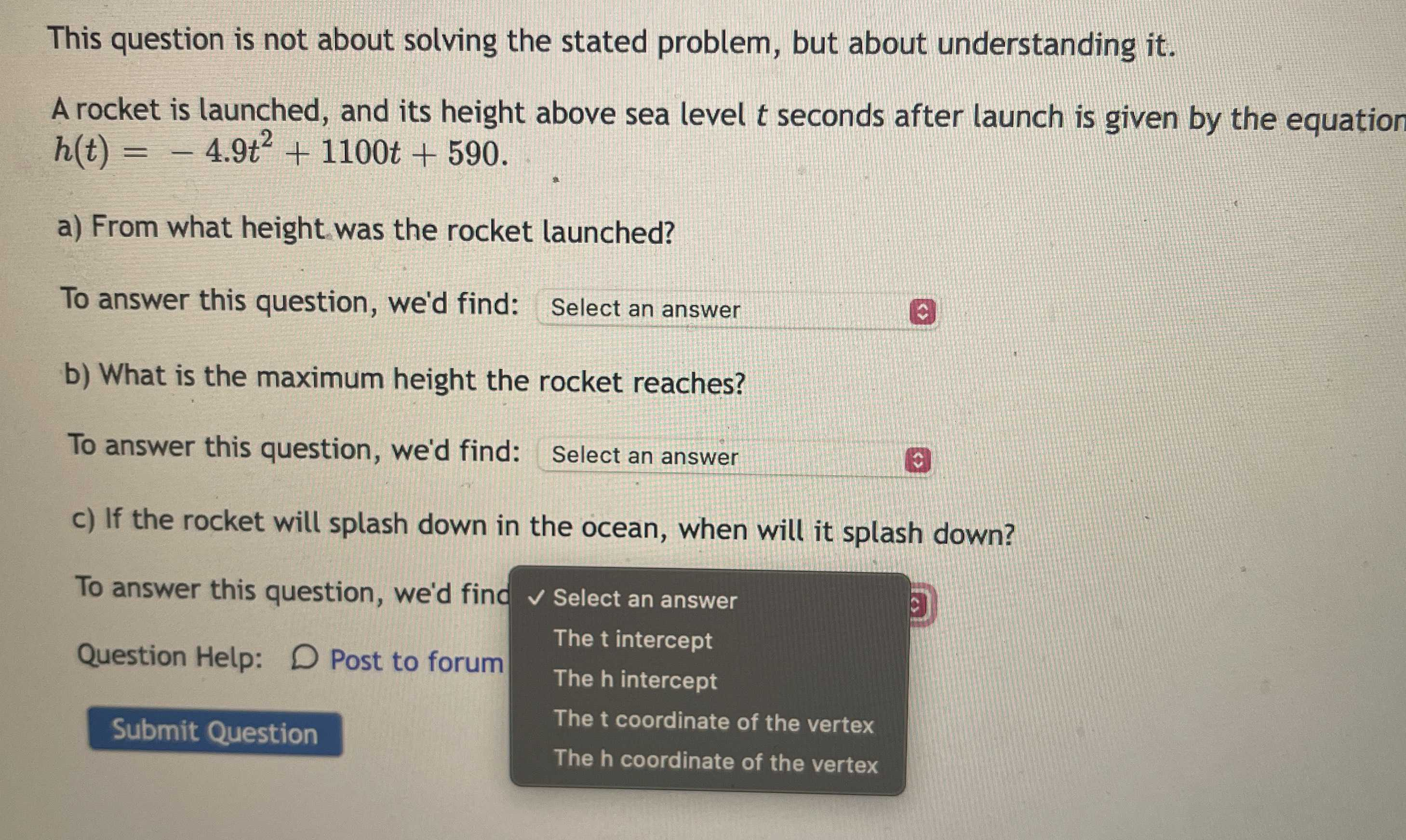### Still have math questions?

Algebra
QuestionA rocket is launched, and its height above sea level $$t$$seconds after launch is given by the equation

$$h ( t ) = - 4.9 t ^ { 2 } + 1100 t + 590$$

a) From what height was the rocket launched?

b) What is the maximum height the rocket reaches?

c) If the rocket will splash down in the ocean, when will it splash down?